# Envelope tracking

Click here to go to our main page on power conditioning circuits

Envelope tracking (ET) is a means of improving power amplifier efficiency and linearity for communication systems.

New for January 2016: Our friends over at Keysight have provided this relevant video that introduces the basic concepts of ET, shows how to generate a shaping table, and concludes with an envelope tracking simulation and results. They'll even let you download an example workspace to run preliminary simulations.

MW101 viewer Andrew sent us most of the content below on envelope tracking.  Thanks, Andrew!

Traditionally, power amplifiers (PAs) are biased so that there is sufficient DC power at the drain of the transistor to supply for peak output RF power conditions. If one is stuck with a single voltage to apply to the drain, it makes sense to choose a voltage which will provide for the peak power. However, when the amplifier is backed-off from peak power the extra DC power must be dissipated in the transistor since it is not being transformed into useful RF power. Supply modulation improves the efficiency of an amplifier by decreasing the supply voltage for backed-off power levels, thus decreasing the dissipated power. The first method of supply modulation was proposed by Kahn and is today known as Envelope Elimination and Restoration (EER); however, we will start our discussion of supply modulation by talking about Envelope Tracking (ET). Figure 1 shows what the power added efficiency (PAE) looks like for a typical power amplifier plotted against output power. In the case of a constant amplitude signal, the amplifier is always operating at its peak efficiency point. When the signal has a high peak-to-average ratio (PAR) and thus varying envelope, the amplifier is forced to operate in less efficient regions, as shown in Figure 2. When a constant amplitude signal is amplified, the PA is always operating at its peak efficiency point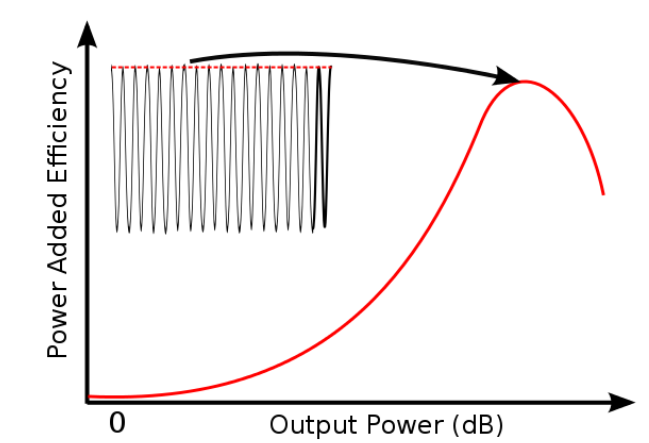Figure 1: Typical efficiency versus output power plot for a power amplifier.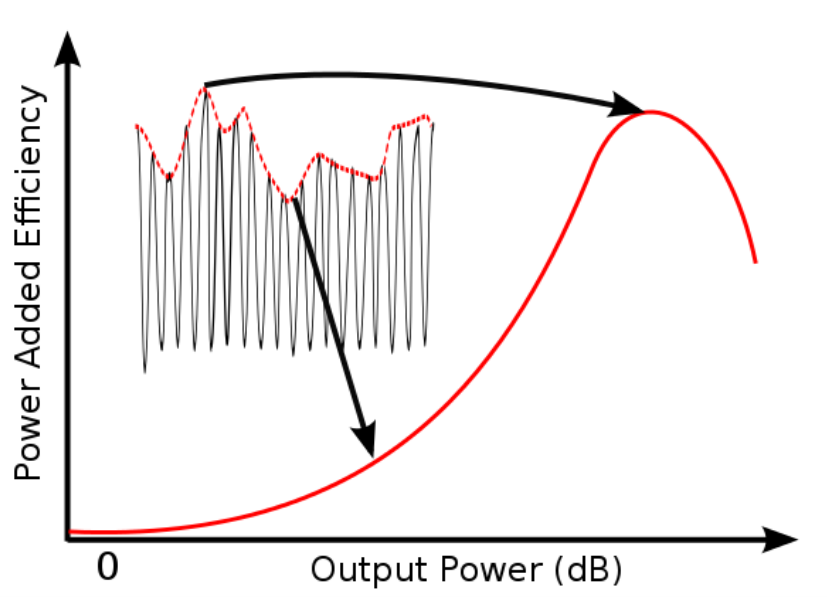Figure 2: If the signal has amplitude modulation, the PA is forced to operate in less efficient regions.

Envelope tracking improves efficiency for backed-off power levels by decreasing the drain voltage. To understand why lowering the drain voltage improves efficiency, consider the IV curves and load line of a Class-A amplifier in Figure 3. Class-A is not an efficient class of operation, but it is beneficial to help explain ET. For a Class-A amplifier to generate the maximum output power for a transistor, it must be biased in the middle of the IV curves so it can swing from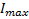to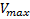, as illustrated by the dashed red line. The best quiescent bias point this case would be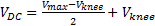and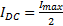. But consider the case when less power is needed from the transistor. The load line for this case is drawn with a solid red line in Figure 3. Even though less RF power is being generated by the transistor, the transistor still has the same quiescent DC bias, and thus dissipates extra DC power than when it is operating at peak RF power.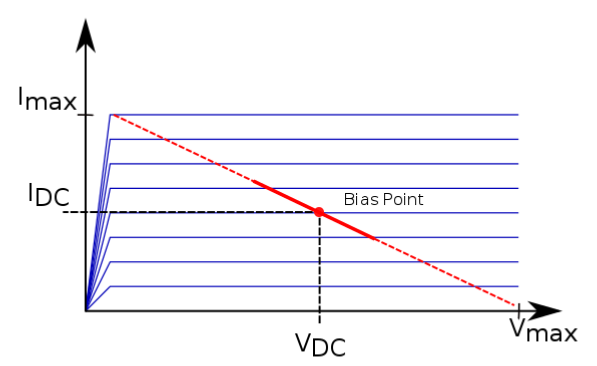Figure 3: A typical power amplifier without supply modulation is biased in the middle of the IV curves so that the transistor is able to swing between the maximum current and voltage values at peak output power (dashed line). However, the bias point remains the same when lower output powers are produced (solid line).

Rather than remain at the optimum peak-power bias point, it is more efficient to adjust the drain supply so that only enough voltage is present to allow for the necessary load line swing, which is what ET does and is shown in Figure 4. In this case, the load line is still able to swing to both of its peaks without saturating or cutting off but operates on less DC supply voltage and therefore consumes less DC power.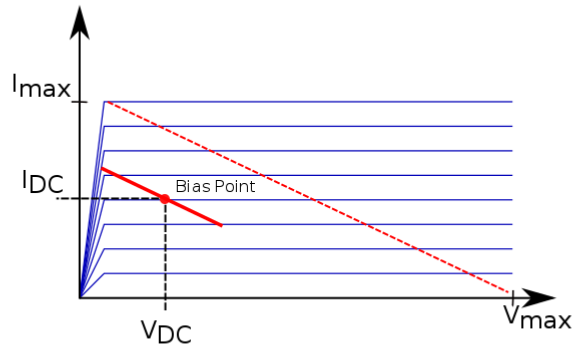Figure 4: Instead of maintaining the same bias point for decreased output power, ET decreases the bias point to allow for enough load line swing while decreasing the DC power.

When the PAE curves are drawn for swept drain voltages, peak efficiency occurs at lower power as shown in Figure 5. Instead of tracing the efficiency of the maximum bias voltage (red curve), envelope tracking allows for the efficiency to follow the peaks of the swept bias voltage, a dramatic improvement. This exercise was shown for Class-A amplifiers, but the same principals apply for other classes of operation.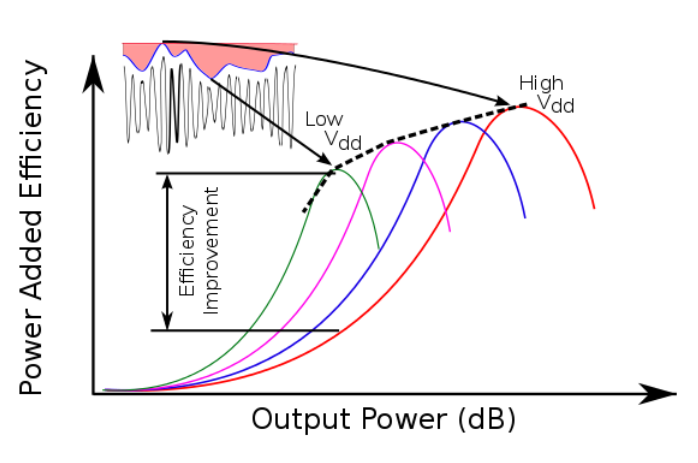Figure 5: When the efficiency curves are plotted vs. output power for stepped supply voltage levels, they resemble the drawing above.

## Envelope elimination and restoration

ET traces the peaks of the stepped efficiency curves instead of following the peak-power bias point efficiency curve (red curve in Figure 5). While ET adjusts the supply level to ensure that extra power is not dissipated, EER uses the supply voltage to shape the output waveform. An input signal with a constant amplitude is applied to the amplifier and the supply level adjusts the gain of the amplifier to shape the output. When Kahn first conceptualized this mode of supply modulation he proposed a Class-C amplifier be used; however, nowadays switched mode amplifiers are typically used. EER requires a highly compressed amplifier and thus is a highly non-linear technique. Envelope tracking on the other hand, while it does not improve the linearity of an amplifier, is certainly more linear than EER.

Co-Author: Andrew Zai

Author : Unknown Editor